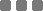## Saturday, January 13, 2018

### Python and JavaScript: How to Create "Manual" Loop Function

In both programming languages, we can find many options for looping. But for this post, we will try to assemble a basic function which can be used to do a "manual" loop using increment. This surely can also use decrement. But for starter, let's use increment.

Concept

First, define the counter index and the limit outside the function as "global" variables.

Then make the function to increment the index. Rerun the function from within it (the function) if the counter hasn't reached the limit we defined.

Sounds really abstract, so let's put concept the coding in. What.

Let's make a function which prints 0 through 5. Like 0 then 1 then 2 ... 5. Without the then.

Python

This is the screenshot from my Python console:

JavaScript

This is the JavaScript console output (on Chrome):

For the JavaScript, actually we don't have to supply the (global) variables (as arguments) to the function, because the variables are defined globally. Hm. But that WON'T WORK in Python. Well, it can definitely work with different typing, for instance, by not defining the parameters, the "using global stuffs without passing things", hm, so behehehe. Because Python is really something, so to make both consistent, I put the JavaScript coding for the function invocation (outside and inside) like the Python's version.

For your information, the difference between parameter and argument: parameter is a function variable used to define the input of that function. Argument is the actual value of the parameter (the actual input value).

With that knowledge, so the function named `loop` above has two parameters, `a` and `b`. And the supplied arguments are defined as (global) variables `e` and `r` (which hold the actual values). I hope that sentence earlier is understandable.

For the decrement (outputting 5 to 0 for example), you just need to switch the `e` into 6 (5 + 1), and the `r` to 0. Then the `if` part is reversed using "greater than" ( > ) operator.
Why "+ 1"? Because first step of that `loop` function is to decrement the index. Therefore, if we define 6 as the initial index, the very first output will be 5.

Similar to the incremental version, -1, because the first step is to increment the index. So when we put -1 to it, the first output will be 0 (-1 + 1 = 0).

Alright let's try to code the decremental "manual" loop.

Python

JavaScript

Well, you can test each one on your own machine.

Restart and Dynamically Modifying the Loop

In the examples above, to restart the loop, set the variable `e` (the initial index) to its initial state, whether -1 or 6. To make it "simpler", we can store the initial index value to a different variable, then assign that initial value (which is stored in a different variable or constant) to the index variable. After that re-initialization, call (invoke) again the `loop` function.

To modify both limits (upper and lower), similar like that restarting method. We can change either one or both dynamically depending what is the trigger (input/limit and the purpose of the loop).

We can also cancel (break) or abort the loop with `if` condition within the function. Again, depends on the trigger(s) we are observing.

Interval

To give an interval between consecutive loop execution, in Python, we can use `time` module. That is `time.sleep(float_in_second)`. For example:

In JavaScript, we can use `window.setTimeout(function..., integer_in_milliseconds)`. For example:

This method is useful to do things in series (as opposed to parallel). For instance, waiting a process to complete, then only after that, move to next item, and so on. Also, we can create another manual loop within it, and within it and within it, and within it, as deep as we need to, but be very extra thorough on defining, using, and passing the variables.

To make it efficient and "easily callable" for different purposes, you could combine all the custom additional stuffs then wrap it into one module (private) function. Uh, that's your own typing and staring at the ceiling time exercise.

And the last, the concept above can always be translated to other languages.Python and JavaScript: How to Create "Manual" Loop Function
https://monkeyraptor.johanpaul.net/2018/01/python-javascript-how-to-create-manual.html